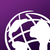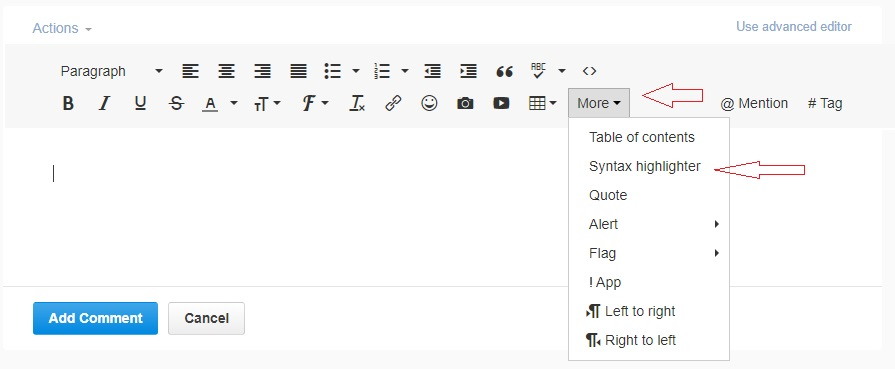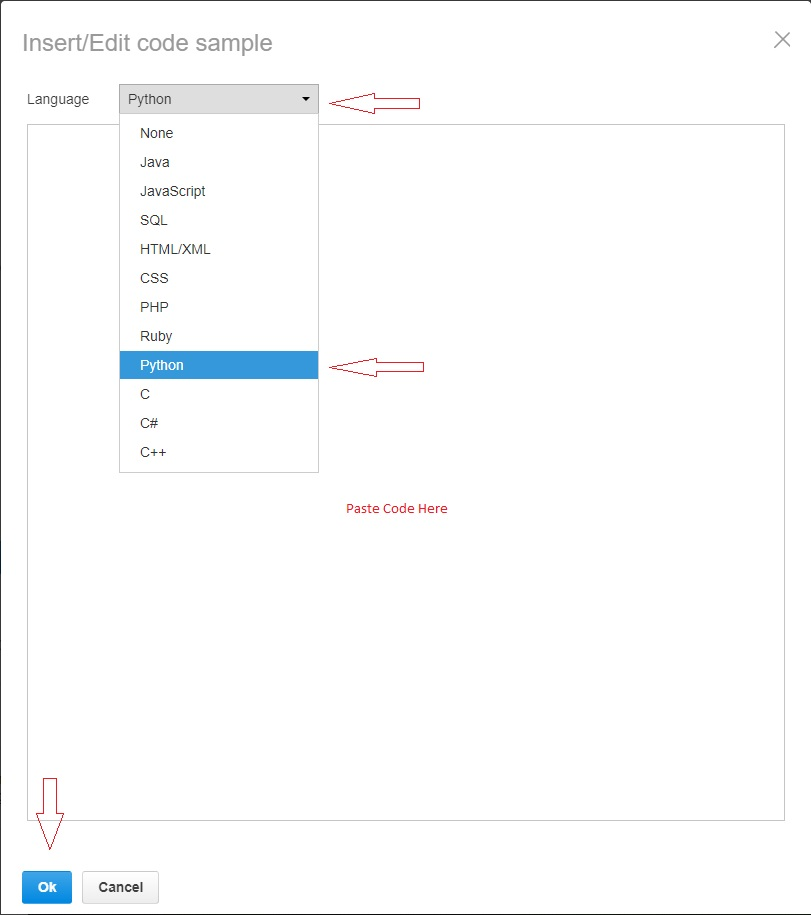# Problem with mask in loop

755
9
12-23-2019 05:46 AMNew Contributor

Hi all.

I have a problem with loop. The script imports the municipalities.shp into ListFeatureClasses. Then the loop inserts them in ExtractByMask 6x. the problem is that the process is done only on fist mask than this two errors apper - Traceback (most recent call last):
File "C:\Users\GBokal\Desktop\scripta_ob.py", line 34, in <module>
File "c:\program files\arcgis\pro\Resources\arcpy\arcpy\sa\Functions.py", line 7337, in ExtractByMask
File "c:\program files\arcgis\pro\Resources\arcpy\arcpy\sa\Utils.py", line 53, in swapper
result = wrapper(*args, **kwargs)
File "c:\program files\arcgis\pro\Resources\arcpy\arcpy\sa\Functions.py", line 7333, in Wrapper
out_raster)
File "c:\program files\arcgis\pro\Resources\arcpy\arcpy\geoprocessing\_base.py", line 506, in <lambda>
return lambda *args: val(*gp_fixargs(args, True))
arcgisscripting.ExecuteError: Failed to execute. Parameters are not valid.
ERROR 000865: Input raster or feature mask data: fc does not exist.
Failed to execute (Script)

or
Traceback (most recent call last):
File "C:\Users\GBokal\Desktop\scripta_ob.py", line 28, in <module>
RuntimeError
Failed to execute (Script).

I am using ArcGIS Pro 2.4.0

My script

``````from arcpy import env
from arcpy.sa import *
from shutil import *
import pathlib
import os
env.overwriteOutput = True
obcine = arcpy.GetParameterAsText(0)
ogro_0 = arcpy.GetParameterAsText(1)
ogro_1 = arcpy.GetParameterAsText(2)
ogro_2 = arcpy.GetParameterAsText(3)
ogro_3 = arcpy.GetParameterAsText(4)
ogro_4 = arcpy.GetParameterAsText(5)
ogro_5 = arcpy.GetParameterAsText(6)
out_folder = arcpy.GetParameterAsText(7) (folder or string path)
arcpy.env.workspace = out_folder
arcpy.env.scratchWorkspace = out_folder
arcpy.FeatureClassToShapefile_conversion(obcine,out_folder)
featureclasses = arcpy.ListFeatureClasses()
for fc in featureclasses:
arcpy.CheckOutExtension("Spatial")
a = str(fc)
b = str(ogro_0)
c = a[:3] + "_" + b[-12:] + (".tif")
out = out_folder + "\\" + c

b = str(ogro_1)
c = a[:3] + "_" + b[-12:] + (".tif")
out = out_folder + "\\" + c

b = str(ogro_2)
c = a[:3] + "_" + b[-12:] + (".tif")
out = out_folder + "\\" + c

b = str(ogro_3)
c = a[:3] + "_" + b[-12:] + (".tif")
out = out_folder + "\\" + c

b = str(ogro_4)
c = a[:3] + "_" + b[-12:] + (".tif")
out = out_folder + "\\" + c

b = str(ogro_5)
c = a[:3] + "_" + b[-12:] + (".tif")
out = out_folder + "\\" + c
arcpy.Delete_management(fc)‍‍‍‍‍‍‍‍‍‍‍‍‍‍‍‍‍‍‍‍‍‍‍‍‍‍‍‍‍‍‍‍‍‍‍‍‍‍‍‍‍‍‍‍‍‍‍‍‍‍‍‍‍‍‍‍‍``````
9 RepliesOccasional Contributor

Gasper Can you please post your code using the syntax highlighter which helps preserve format and indentation. To do this click the 3 dots:Then click more and choose Syntax Highlighter:Then choose Python from the language drop down menu, paste your code and click OkbyMVP Legendary Contributor

or save the bookmarkbyEsri Regular Contributor

...one of the reasons for doing this is to rule out the obvious;

ERROR 000865: Input raster or feature mask data: fc does not exist. is thrown

and the last statement is

arcpy.Delete_management(fc)New Contributor

Unfortunately, it is not a problem in the arcpy.Delete_management(fc). The loop stops at the second mask

``outExtractByMask = ExtractByMask(ogro_1, fc)``

the first one is completed as expected.byMVP Legendary Contributor

throw in print statements to see what values you have for the parameters, and correct Arne Gelfert‌ suggestion for sureOccasional Contributor III

Did you forget to comment out part of this line?

``out_folder = arcpy.GetParameterAsText(7) (folder or string path)‍‍``

Maybe...

``out_folder = arcpy.GetParameterAsText(7) # (folder or string path)‍‍``New Contributor

Sorry for the late reply it was a long holiday. If I insert print() statement I get all the correct paths as I wanted.

``````from arcpy import env
from arcpy.sa import *
from shutil import *
import pathlib
import os

env.overwriteOutput = True

obcine = arcpy.GetParameterAsText(0)
ogro_0 = r"D:\GH-15\podatki_apk.gdb\Obala_ogroženost_0"
ogro_1 = r"D:\GH-15\podatki_apk.gdb\Obala_ogroženost_1"
ogro_2 = r"D:\GH-15\podatki_apk.gdb\Obala_ogroženost_2"
ogro_3 = r"D:\GH-15\podatki_apk.gdb\Obala_ogroženost_3"
ogro_4 = r"D:\GH-15\podatki_apk.gdb\Obala_ogroženost_4"
ogro_5 = r"D:\GH-15\podatki_apk.gdb\Obala_ogroženost_5"
out_folder = "C:\\Users\\GBokal\\Desktop\\scripta"
arcpy.env.workspace = out_folder
arcpy.env.scratchWorkspace = out_folder
arcpy.FeatureClassToShapefile_conversion(obcine,out_folder)
featureclasses = arcpy.ListFeatureClasses()

for fc in featureclasses:
print(fc)
arcpy.CheckOutExtension("Spatial")
a = str(fc)
b = str(ogro_0)
c = a[:3] + "_" + b[-12:] + (".tif")
print(c)
out0 = os.path.join(out_folder,c)
print(out0)

results
izola_buff.shp
izo_ogroženost_0.tif
C:\Users\GBokal\Desktop\scripta\izo_ogroženost_0.tif
koper_buff.shp
kop_ogroženost_0.tif
C:\Users\GBokal\Desktop\scripta\kop_ogroženost_0.tif
piran_buff.shp
pir_ogroženost_0.tif
C:\Users\GBokal\Desktop\scripta\pir_ogroženost_0.tif‍‍‍‍‍‍‍‍‍‍‍‍‍‍‍‍‍‍‍‍‍‍‍‍‍‍‍‍‍‍‍‍‍‍‍‍‍‍‍‍‍‍‍‍‍‍‍‍‍‍‍‍‍‍‍‍‍‍‍‍‍‍‍‍‍‍‍‍‍‍‍‍‍‍‍‍‍‍‍‍``````

But as soon as I put the ExtractByMask statement the loop stops at first loop.

``````from arcpy import env
from arcpy.sa import *
from shutil import *
import pathlib
import os

env.overwriteOutput = True

obcine = arcpy.GetParameterAsText(0)
ogro_0 = r"D:\GH-15\podatki_apk.gdb\Obala_ogroženost_0"
ogro_1 = r"D:\GH-15\podatki_apk.gdb\Obala_ogroženost_1"
ogro_2 = r"D:\GH-15\podatki_apk.gdb\Obala_ogroženost_2"
ogro_3 = r"D:\GH-15\podatki_apk.gdb\Obala_ogroženost_3"
ogro_4 = r"D:\GH-15\podatki_apk.gdb\Obala_ogroženost_4"
ogro_5 = r"D:\GH-15\podatki_apk.gdb\Obala_ogroženost_5"
out_folder = "C:\\Users\\GBokal\\Desktop\\scripta"
arcpy.env.workspace = out_folder
arcpy.env.scratchWorkspace = out_folder
arcpy.FeatureClassToShapefile_conversion(obcine,out_folder)
featureclasses = arcpy.ListFeatureClasses()

for fc in featureclasses:
print(fc)
arcpy.CheckOutExtension("Spatial")
a = str(fc)
b = str(ogro_0)
c = a[:3] + "_" + b[-12:] + (".tif")
print(c)
out0 = os.path.join(out_folder,c)
print(out0)

#results
izola_buff.shp
izo_ogroženost_0.tif
C:\Users\GBokal\Desktop\scripta\izo_ogroženost_0.tif
koper_buff.shp
kop_ogroženost_0.tif
C:\Users\GBokal\Desktop\scripta\kop_ogroženost_0.tif

#C:\Users\GBokal\Desktop\scripta\kop_ogroženost_0.tif was not created in out_folder.‍‍‍‍‍‍‍‍‍‍‍‍‍‍‍‍‍‍‍‍‍‍‍‍‍‍‍‍‍‍‍‍‍‍‍‍‍‍‍‍‍‍‍‍‍‍‍‍‍‍‍‍‍‍‍‍‍‍‍‍‍‍‍‍‍‍‍‍‍‍‍‍‍‍‍‍‍‍‍‍‍‍‍‍``````

I am running out of ideas on how to fix the script.byMVP Legendary Contributor

Gasper, I am not seeing the full path to the input featureclass and the input tif, It could be the workspace assumption being correct that is the issue, so concatenate the full path name to them.

Also, if it works manually through the arctoolbox interface you can export the tool outputs to a python script and examine it for the 'hidden obvious'Occasional Contributor

Since you are getting the "FC doesn't exist" error, I took a look at the variables. So with your "for" statement you are trying to iterate through the "featureclasses" variable which is set to arcpy.ListFeatureClasses() which would be trying to get the featureclasses from within the workspace. However I noticed that your workspace might be getting set to a folder. Try setting your workspace to a geodatabase and see if the script runs.

Disregard this reply. I just saw where you said the script stops at the second mask, which means arcpy.ListFeatureClasses() is getting populated and is working with the current workspace.